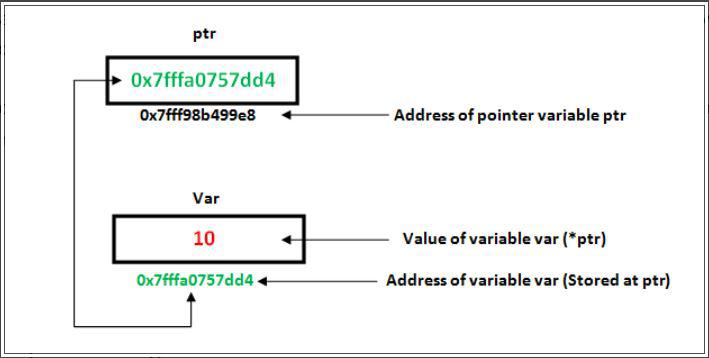GFG App
Open AppBrowser
Continue

# C++ Pointer Operators

Prerequisite: Pointers in C++

A pointer variable is a variable that stores the address of another variable or in other a pointer variable points to the variable whose address is stored inside it.

Syntax:

`int *pointer_name; `

There are mainly two types of Pointer operators mainly used:

• The indirection operator/Dereference operator (*)Image showing the relation between pointer and variable

The Address-of operator (&) is a unary operator that returns the memory address of its operand which means it stores the address of the variable, which depicts that we are only storing the address not the numerical value of the operand. It is spelled as the address of the variable.

Syntax:

`gfg = &x; // the variable gfg stores the address of the variable x.`

Example:

## C++

 `// C++ Program to demonstrate` `// the use of Address-of operator (&)` `#include ` `using` `namespace` `std;`   `int` `main()` `{`   `    ``int` `x = 20;`   `    ``// Pointer pointing towards x` `    ``int``* ptr = &x;`   `    ``cout << ``"The address of the variable x is :- "` `<< ptr;` `    ``return` `0;` `}`

Output

`The address of the variable x is :- 0x7fff412f512c`

## 2. The indirection operator/Dereference operator (*)

The indirection/ dereference operator is a unary operator that returns the value of the variable present at the given address. It is completely opposite to the address-of operator. It is spelled as a value pointed at the address.

Example:

## C++

 `// C++ Program  to Demonstrate` `// the indirection/Dereference operator` `#include ` `using` `namespace` `std;`   `int` `main()` `{`   `    ``int` `self_paced = 3899;` `    ``int``* price;`   `    ``// Pointer storing address of self_paced` `    ``price = &self_paced;`   `    ``cout` `        ``<< ``"The value stored at the variable price is Rs : "` `        ``<< (*price);`   `    ``return` `0;` `}`

Output

`The value stored at the variable price is Rs : 3899`

My Personal Notes arrow_drop_up